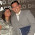## Monday, January 3, 2011

### Godspeed

One day I was sitting in my Physics 106 class at BYU, learning about Einstein's Theory of Special Relativity. The teacher was explaining the effects of near-light travel and giving us equations to define each. When he mentioned time dilation :
ΔTmoving = ΔT­rest √(1 – v2/c2)
I wondered: could I use this to figure out how fast God is moving?

In Psalm 90:4 it says that 1000 years for man is are 1 day for God. For our purposes, we will assume we are stationary. This yields:
1 d = 1000 yr √(1 – v2 / c2)
To convert years into days, we multiply by 365:
1 d = 365,000 d √(1 – v2 / c2)
We divide both sides by 365,000:
1 / 365,000 = √(1 – v2 / c2)
The velocity (v) will be a fraction of the speed of light (c), so we can define v in terms of c, where x is the percentage of c:
v = xc
Substituting that in, we get:
1 / 365,000 = √(1 – x2c2 / c2)
This allows us to cancel out c, leaving us with:
1 / 365,000 = √(1 – x2)
We square both sides:
(1 / 365,000)2 = 1 – x2
We rearrange to get x2 by itself:
x2 = 1 – (1 / 365,000)2
To simplify, we take the square root of both sides:
x = √(1 – (1 / 365,000)2)
We solve for x (which gives us the percentage of the speed of light):
x = 0.999 999 999 996 247
We substitute x back into v = xc:
v = 0.999 999 999 996 247c
We substitute 299,792,458 m/s for c:
v = 0.999 999 999 996 247 × 299,792,458 m/s 
And solve, to find the velocity of God:
v = 299,792,457.988 874 878 905 126 m/s

Notes:

 Time dilation is the phenomenon where your perception of the passage of time is altered as you approach the speed of light. In other words, time passes more slowly at near-light speeds than it does for a stationary observer. So if you were to fly to Aldebaran at 0.99% of light speed and then immediately fly back to the Solar System, it would take you roughly 18.3 years. But when you got back you'd learn that approximately 130 years had passed on Earth since you left and everyone you knew was long dead.

 Compare 2 Peter 3:8, which posits a paradoxical interconversion, and Abraham 3:4, which offers an astronomical, rather than relativistic, rationale.

 In fact, we are not stationary. The earth is moving at ~30 km/s around the Sun, the solar system is moving at ~220 km/s around the galactic center, and the Milky Way is moving at ~600 km/s through space.

 It is interesting to compare the idea that God is moving at or near light speed to the following Scriptural passages: 1 John 1:5; D&C 6:21; D&C 11:28; and D&C 88:5–13, 50.

Albert Einstein is by Yousuf Karsh, posted by Père Ubu at http://www.flickr.com/photos/8001758@N02/3027907814.

1.I have two questions. 1) When Psalms was written wasn't it using the Hebrew year which was lunar and not 365 days? 2) Ignoring the last question; if we're using our year wouldn't the conversion be 365.25*1,000? or 365,250 days? (Or would the additional 250 days be insignificant to the outcome?)

Anyway, this is one of those math problems that is always fun to calculate. Another is using the 1 day = 1,000 year to figure out how long you've been alive. I think I heard once that it's less than five minutes.

2.Quiero este en mi blog también mi estimado mateo crook, espero puedas ver mis mensajes ya que no tengo tu correo electrónico.

Saludos

3.